## Пятница 09.12. Galina Jiraskova: " Self-Verifying Finite Automata"

Пятница, 9 декабря, ауд. 311. Начало в 16:00.

Докладчик: Galina Jiraskova (Mathematical Institute Slovak Academy of Sciences).

Тема: Self-Verifying Finite Automata.

### Abstract

Consider a nondeterministic finite automaton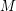over an alphabet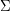,
where the states can be either accepting, rejecting, or neutral. Let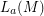(resp.,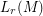) be the set of strings takingfrom the start state to an accepting state (resp., rejecting state). Thenis said to be self-verifying
if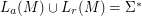and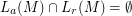.
That is, the strings reaching an accepting state and the strings reaching a rejecting state form a disjoint partition of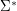. The language accepted byis.

Using a result of Moon, Moser [On cliques in graphs, Israel J. Math. 3, 23--28, 1965], we get an optimal simulation of self-verifying automata by deterministic finite automata. Then we study the complexity of regular operations on languages represented by self-verifying automata, and get the tight upper bounds for union (), intersection (), reversal (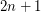), star (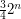), left quotient (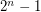), right quotient (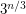), and asymptotically tight upper bound for concatenation (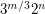). Next we prove that given a nondeterministic automatonwith accepting, rejecting, and neutral states, it is PSPACE-complete to determine ifis self-verifying. Finally, we study the complexity of the membership, emptiness, universality, and minimization problems for self-verifying automata.